# TensorFlow从1到2（十二）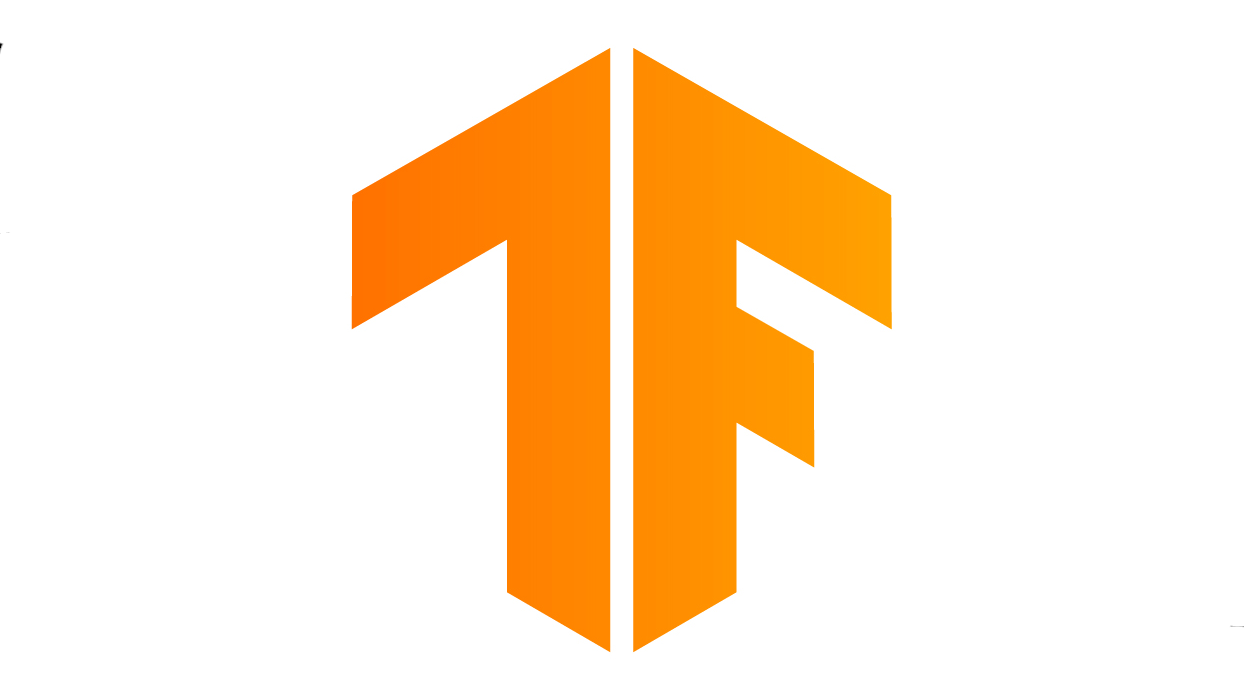#### 生成对抗网络的概念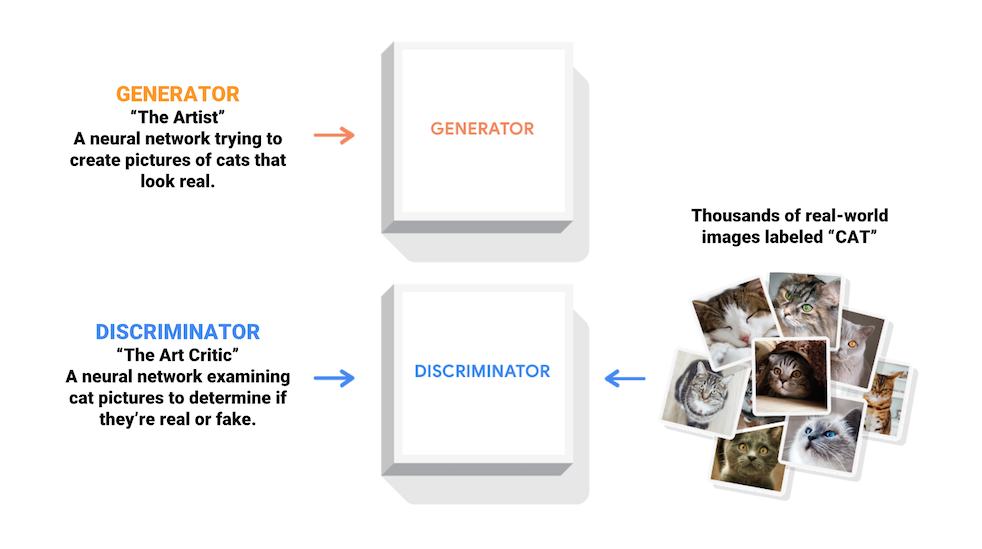(图片来自官方文档)
“考官”负责检查样本和生成图。这里有一个区别于VAE模型的重点，VAE是直接比较样本和生成图，以两者的差距作为代价。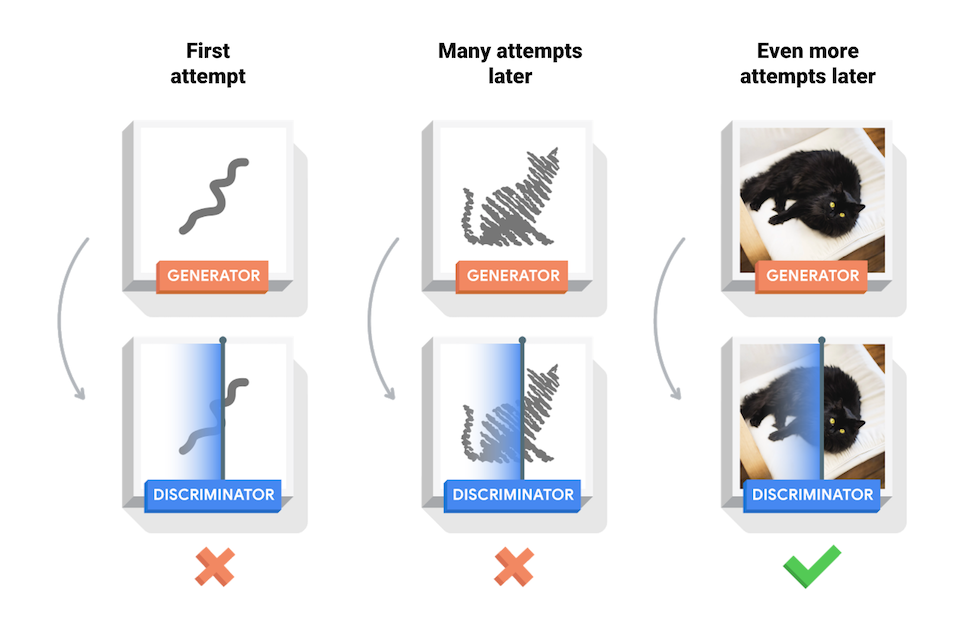（图片来自官方文档）

#### GAN实例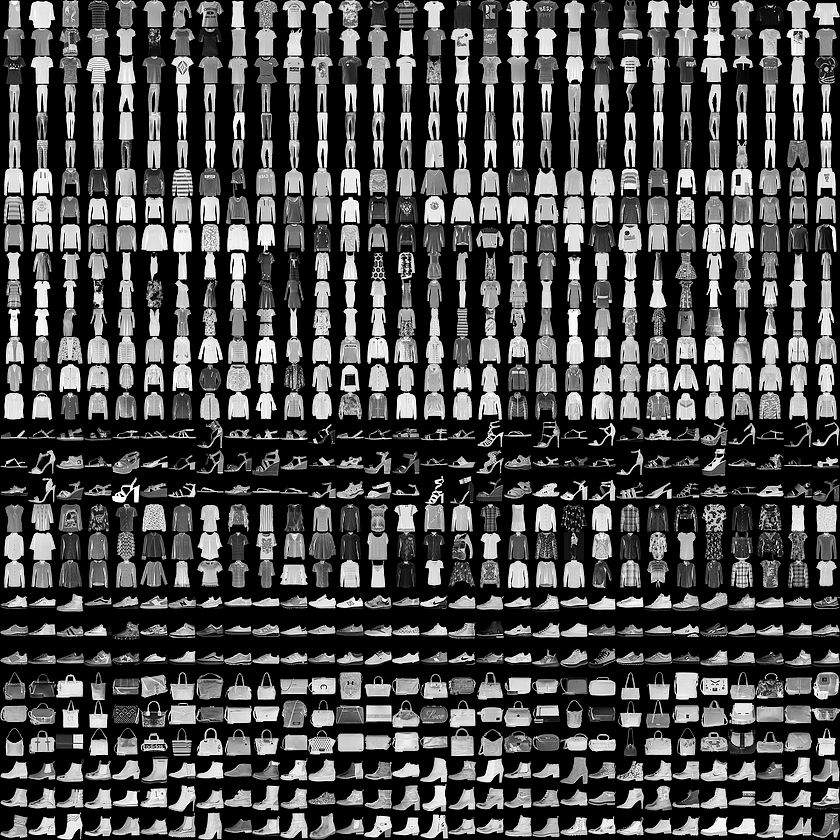``````(train_images, _), (_, _) = keras.datasets.fashion_mnist.load_data()
``````

``````    ...假设本层输入为7x7x256...
layers.Conv2DTranspose(128, (5, 5), strides=(1, 1), padding='same', use_bias=False))
...因为节点数为128，步长是1...
...所以输出维度是7x7x128...
layers.Conv2DTranspose(64, (5, 5), strides=(2, 2), padding='same', use_bias=False))
...输出维度为14x14x64...
``````

``````# 生成模型的损失函数
def generator_loss(fake_output):
# 生成模型期望最终的结果越来越接近1，也就是真实样本
return cross_entropy(tf.ones_like(fake_output), fake_output)
``````

``````# 辨别模型损失函数
def discriminator_loss(real_output, fake_output):
# 样本图希望结果趋近1
real_loss = cross_entropy(tf.ones_like(real_output), real_output)
# 自己生成的图希望结果趋近0
fake_loss = cross_entropy(tf.zeros_like(fake_output), fake_output)
# 总损失
total_loss = real_loss + fake_loss
``````

#### 完整源码

``````#!/usr/bin/env python3

from __future__ import absolute_import, division, print_function, unicode_literals
import tensorflow as tf
from tensorflow import keras
import glob
import imageio
import matplotlib.pyplot as plt
import numpy as np
import os
import PIL
from tensorflow.keras import layers
import time
import sys

# 如果使用train参数运行则进入训练模式
TRAIN = False
if len(sys.argv) == 2 and sys.argv == 'train':
TRAIN = True

# 使用手写字体样本做训练
# (train_images, _), (_, _) = keras.datasets.mnist.load_data()
# 使用时尚单品样本做训练
(train_images, _), (_, _) = keras.datasets.fashion_mnist.load_data()

# 因为卷积层的需求，增加色深维度
train_images = train_images.reshape(train_images.shape, 28, 28, 1).astype('float32')
# 规范化为-1 - +1
train_images = (train_images - 127.5) / 127.5

BUFFER_SIZE = 60000
BATCH_SIZE = 256
train_dataset = tf.data.Dataset.from_tensor_slices(train_images).shuffle(BUFFER_SIZE).batch(BATCH_SIZE)

# 图片生成模型
def make_generator_model():
model = tf.keras.Sequential()

assert model.output_shape == (None, 7, 7, 256) # Note: None is the batch size

assert model.output_shape == (None, 7, 7, 128)

assert model.output_shape == (None, 14, 14, 64)

assert model.output_shape == (None, 28, 28, 1)

return model

generator = make_generator_model()

# 原图、生成图辨别网络
def make_discriminator_model():
model = tf.keras.Sequential()
input_shape=[28, 28, 1]))

return model

discriminator = make_discriminator_model()

# 随机生成一个向量，用于生成图片
noise = tf.random.normal([1, 100])
# 生成一张，此时模型未经训练，图片为噪点
generated_image = generator(noise, training=False)
# plt.imshow(generated_image[0, :, :, 0], cmap='gray')
# 判断结果
decision = discriminator(generated_image)
# 此时的结果应当应当趋近于0，表示为伪造图片
print(decision)

# 交叉熵损失函数
cross_entropy = tf.keras.losses.BinaryCrossentropy(from_logits=True)

# 辨别模型损失函数
def discriminator_loss(real_output, fake_output):
# 样本图希望结果趋近1
real_loss = cross_entropy(tf.ones_like(real_output), real_output)
# 自己生成的图希望结果趋近0
fake_loss = cross_entropy(tf.zeros_like(fake_output), fake_output)
# 总损失
total_loss = real_loss + fake_loss

# 生成模型的损失函数
def generator_loss(fake_output):
# 生成模型期望最终的结果越来越接近1，也就是真实样本
return cross_entropy(tf.ones_like(fake_output), fake_output)

# 训练结果保存
checkpoint_dir = 'dcgan_training_checkpoints'
checkpoint_prefix = os.path.join(checkpoint_dir, "ckpt")
checkpoint = tf.train.Checkpoint(generator_optimizer=generator_optimizer,
discriminator_optimizer=discriminator_optimizer,
generator=generator,
discriminator=discriminator)

EPOCHS = 100
noise_dim = 100
num_examples_to_generate = 16

# 初始化16个种子向量，用于生成4x4的图片
seed = tf.random.normal([num_examples_to_generate, noise_dim])

# @tf.function表示TensorFlow编译、缓存此函数，用于在训练中快速调用
@tf.function
def train_step(images):
# 随机生成一个批次的种子向量
noise = tf.random.normal([BATCH_SIZE, noise_dim])

# 生成一个批次的图片
generated_images = generator(noise, training=True)

# 辨别一个批次的真实样本
real_output = discriminator(images, training=True)
# 辨别一个批次的生成图片
fake_output = discriminator(generated_images, training=True)

# 计算两个损失值
gen_loss = generator_loss(fake_output)
disc_loss = discriminator_loss(real_output, fake_output)

# 根据损失值调整模型的权重参量

# 计算出的参量应用到模型

def train(dataset, epochs):
for epoch in range(epochs+1):
start = time.time()

for image_batch in dataset:
train_step(image_batch)

# 每个训练批次生成一张图片作为阶段成功
print("=======================================")
generate_and_save_images(
generator,
epoch + 1,
seed)

# 每20次迭代保存一次训练数据
if (epoch + 1) % 20 == 0:
checkpoint.save(file_prefix=checkpoint_prefix)

print('Time for epoch {} is {} sec'.format(epoch + 1, time.time()-start))

def generate_and_save_images(model, epoch, test_input):
# 设置为非训练状态，生成一组图片
predictions = model(test_input, training=False)

fig = plt.figure(figsize=(4,4))

# 4格x4格拼接
for i in range(predictions.shape):
plt.subplot(4, 4, i+1)
plt.imshow(predictions[i, :, :, 0] * 127.5 + 127.5, cmap='gray')
plt.axis('off')

# 保存为png
plt.savefig('image_at_epoch_{:04d}.png'.format(epoch))
# plt.show()
plt.close()

# 遍历所有png图片，汇总为gif动图
def write_gif():
anim_file = 'dcgan.gif'
with imageio.get_writer(anim_file, mode='I') as writer:
filenames = glob.glob('image*.png')
filenames = sorted(filenames)
last = -1
for i, filename in enumerate(filenames):
frame = 2*(i**0.5)
if round(frame) > round(last):
last = frame
else:
continue
writer.append_data(image)
writer.append_data(image)

# 生成一张初始状态的4格图片，应当是噪点
generate_and_save_images(
generator,
0000,
seed)

if TRAIN:
# 以训练模式运行，进入训练状态
train(train_dataset, EPOCHS)
write_gif()
else:
# 非训练模式，恢复训练数据
checkpoint.restore(tf.train.latest_checkpoint(checkpoint_dir))
print("After training:")
# 显示训练完成后，生成图片的辨别结果
generated_image = generator(noise, training=False)
decision = discriminator(generated_image)
# 结果应当趋近1
print(decision)
# 重新生成随机值，生成一组图片保存
seed = tf.random.normal([num_examples_to_generate, noise_dim])
generate_and_save_images(
generator,
9999,
seed)
``````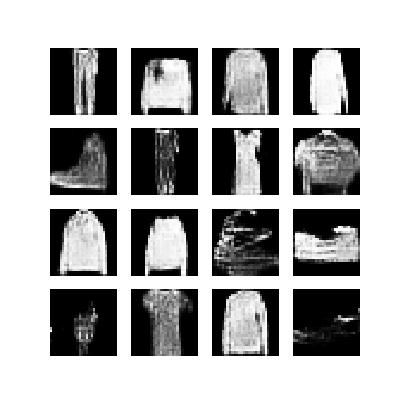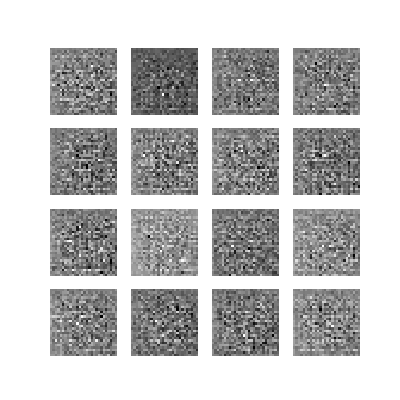在所有模型未经训练的时候，我们随机生成了一幅图片，使用辨别器进行了判断。在训练完成之后，我们再次重复这一过程。通过命令行的输出，我们可以看到类似这样的结果：

``````tf.Tensor([[-4.8871705e-05]], shape=(1, 1), dtype=float32)
After training:
tf.Tensor([[-1.5235078]], shape=(1, 1), dtype=float32)
``````

（待续…）